# What is this Crest Factor Thing?

#### Tech Note

In the early days of electrification, Edison distributed DC electric power. Tesla and Westinghouse had a better idea, AC. There was, and is, a need to understand the equivalence of the two. The most basic unit of interest is power measured in Watts. A Watt is a measure of the flow of energy in joules/second. The utility company billing unit is the kilowatthour, or 3,600,000 joules.

Wattage represents heating value. 100Volts DC into a 100Ohm resistor is 1Amp, and 100Volts x 1Amp = 100Watts. What AC value of voltage and current deliver 100Watts energy flow to the resistor? Call these Vrms and Irms, so 100Vrms x 1Arms = 100Watts. As they say in textbooks, “it can be shown” that the rms, or effective value called root mean square, of any periodic function is given by the following formula where T is the period.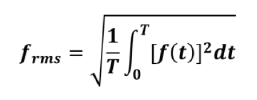All is well with AC sine waves, but current, and sometimes voltage, become distorted by everyday loads. For example, the common full wave, diode and capacitor rectifier converts AC to DC for semiconductor circuits. The capacitor filter and its parallel load take current in pulses somewhat as in following figure. The diodes only turn on for a small portion of the voltage cycle. Source current limiting, diode characteristics, and capacitor characteristics would make the actual wave forms more complicated than shown.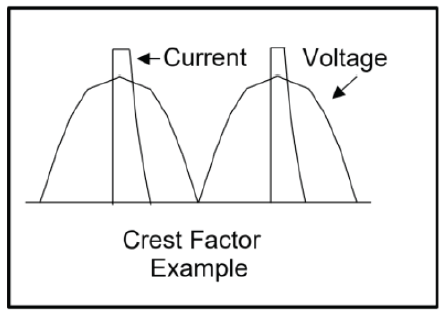What is commonly called crest factor is defined as C in the next formula.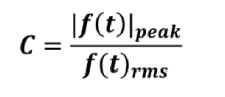This factor is necessary to characterize any electronic method used to produce a linear output proportional to rms value. One common method is a processor and analog to digital converter (ADC) to sample at points on the curves and make the calculation numerically. A second is special circuits of transistors. Both of these have limits. The first is limited by sampling and computation time; although current devices can easily handle 60Hz. The second is limited by the bandwidth and stability of transistor circuits. Both methods are more accurate for lower crest factors and become less accurate as the crest factor increases. Thus, a system or device can be rated for a given accuracy below a given crest factor. Another term is peak to average power ratio defined in the following formula,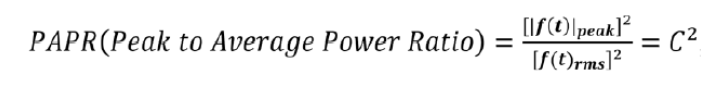Or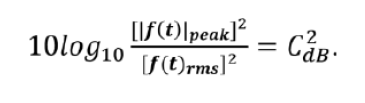Top